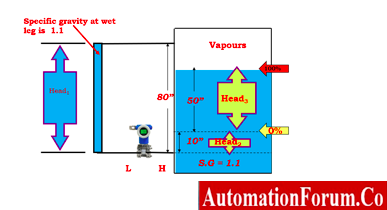# Open and Closed Tank DP Level Calculations## Open Vessel Level Measurement Calculation

### a) Example calculation for the transmitter installed in zero-based direct mount application

The Calibration Range may be computed using the following method:

At zero percentage of level (for 4mA output)

Pressure = 1.1 X 0 (head level is 0”)

At 4 mA = 0”H2O

At 100 percentage of level (for 20mA output)

Pressure = 1.1 X 80”(head level is 80”)

At 20mA= 88 ”H2O

The calibration range of the transmitter is  = 0 to 88”H2O(4 to 20mA)

### b) Example calculation for the transmitter installed in non- zero-based direct mount application

The Calibration Range may be computed using the following method:

At zero percentage of level (for 4mA output)

= 1.1 X 10”

At 4 mA  = 11”H2O

At 100 percentage of level (for 20mA output)

= 1.1 x 70”

At 20 mA = 77 ”H2O

So the calibration range of the transmitter is  = 11’’H2O  to 77”H2O(4 to 20mA)

### c) Example calculation for the transmitter installed in non- zero-based remote mount application

The Calibration Range may be computed using the following method:

At zero percentage of level (for 4mA output)

= 1.1 X 10”

At 4 mA= 11”H2O

At 100 percentage of level (for 20mA output)

= (1.1 X 10”) + (1.1 X 80”)

=11” + 88’’

At 20 mA= 99 ”H2O

So the calibration range of the transmitter is  = 11’’H2O  to 99”H2O(4 to 20mA)

## Closed Tank Level Calculation with Wet Leg

### a) Example calculation for the transmitter installed in zero based direct mount application

The Calibration Range for the DP transmitter is calculated as like below

At zero percentage of level (for 4mA output)

Differential pressure   = Phigh – Plow

= 0 – (1.1 X 80”)

At 4 mA= -88”H2O

At 100 percentage of level (for 20mA output)

Differential pressure   = Phigh – Plow

= (1.1 X 60”)] – (1.1 x 80”)

=66-88

At 20 mA = -22”H2O

Cal. Range = -88 to -22”H2O(4 to 20mA)

### b) Example calculation for the transmitter installed in non zero based direct mount application,

The Calibration Range for the DP transmitter is calculated as like below

At zero percentage of level (for 4mA output)

DP   = Phigh – Plow

= (1.1 X 10”) – (1.1 X 80”)

=11” – 88”

At 4 mA = -77”H2O

At 100 percentage of level (for 20mA output)

DP   = Phigh – Plow

= [(1.1 X 10”) + (1.1 X 50”)] – (1.1 X 80”)

=11”+55”-88”

At 20 mA  = -22”H2O

Cal. Range = -77 to -22”H2O(4 to 20mA)

### c) Example calculation for the transmitter installed in non zero based remote mount application

The Calibration Range for the DP transmitter is calculated as like below

At zero percentage of level (for 4mA output)

DP   = Phigh – Plow

= (1.1 X 10”) – (1.1 X 90”)

=11” – 99”

At 4 mA  = -88”H2O(LRV)

At 100 percentage of level (for 20mA output)

DP   = Phigh – Plow